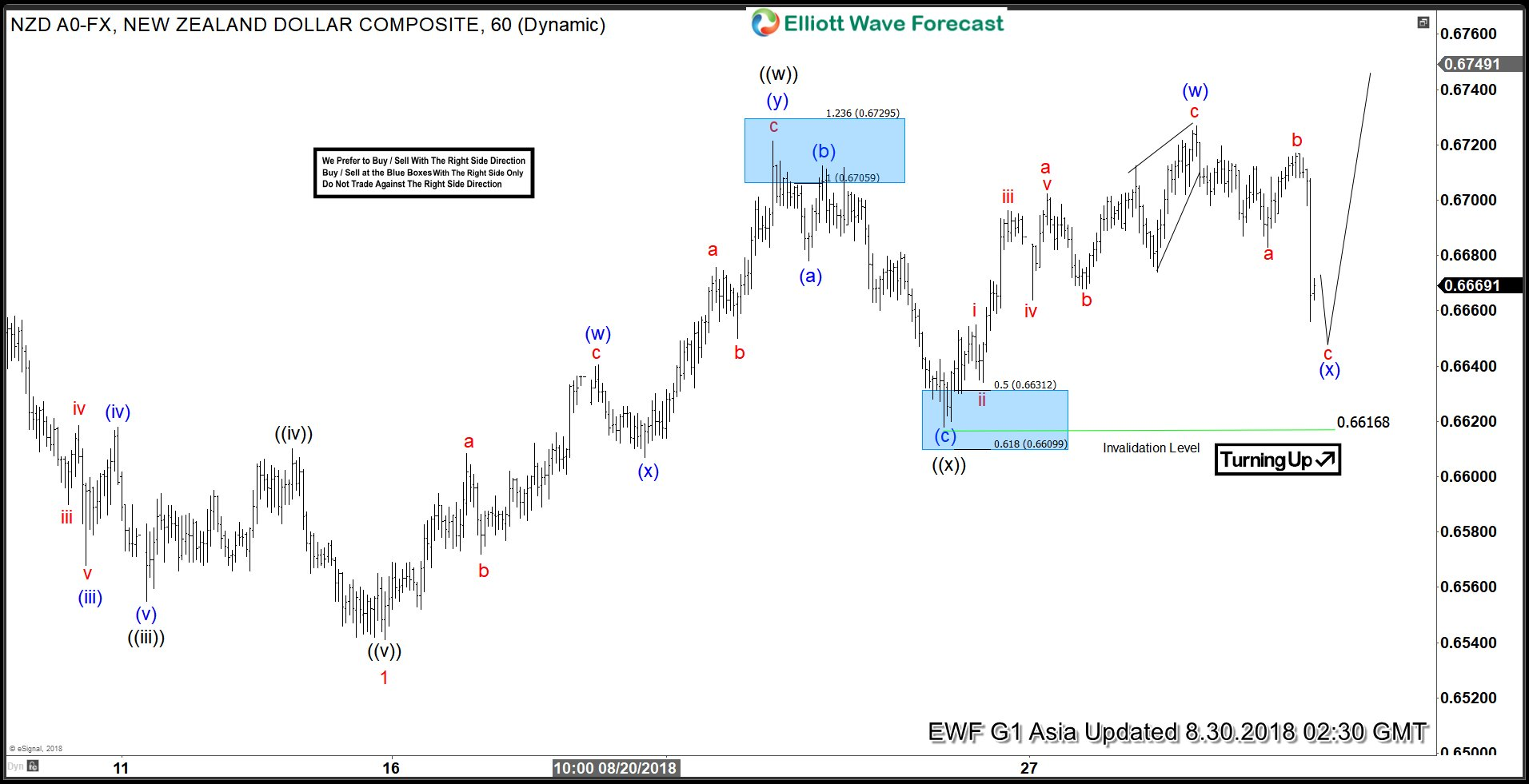# NZDUSD Elliott Wave Analysis: Double Correction Taking Place

NZDUSD short-term elliott wave analysis suggests that the decline to 0.6543 low ended Minor wave 1. The internals of that decline unfolded as impulse structure with lesser degree Minute wave ((i)), ((iii)) & ((v)) unfolded in 5 waves structure. Above from there, Minor wave 2 bounce is taking place as double correction higher with lesser degree cycles showing sub-division of 3 wave structure its each leg higher.

Up from 0.6543 low, the rally to 0.6719 high ended Minute wave ((w)) of 2. The internals of that rally also unfolded as double three structure where Minutte degree wave (w) ended at 0.6639. Minutte wave (x) pullback ended at 0.6609 & Minutte (y) of ((w)) ended at 0.6719 high. From there, the pullback to 0.6616 low ended Minute wave ((x)) of 2 as a Flat structure. Near-term, as far as dips remain above there, expect pair to resume the next leg higher in Minute wave ((y)) of 2 looking for 0.6795-0.6835, which is the 100%-123.6% Fibonacci extension area of Minute wave ((w))-((x)) to end Minor wave 2 bounce. We don’t like buying the pair.

#### NZDUSD 1 Hour Elliott Wave Chart